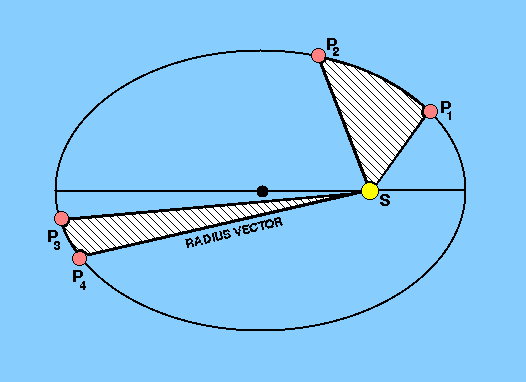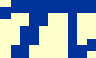Kepler also investigated the speeds of the planets and found that the closer in its orbit a planet is to the Sun, the faster it moves. Drawing a straight line connecting the Sun and the planet (the radius vector), he discovered that he could express this fact in his second law - the law of areas:

For any planet the radius vector sweeps out equal areas in equal times.

 figure 32: Kepler's second law.Figure 32 shows the positions of a planet, P1, P2, P3, P4, at times t1, t2, t3, t4. Between times t1 and t2 the planet's radius vector sweeps out the area bounded by the radius vectors SP1, SP2 and the arc P1P2. Similarly, the area swept out by the radius vector in the time interval (t4-t3) is the area SP3P4. Then, Kepler's second law states that:

area SP1P2 / (t2-t1) = area SP3P4 / (t4-t3) = constant.

If t2-t1 = t4-t3, then area SP1P2 = area SP3P4.

If the area is the area of the ellipse itself (which is given byab), the radius vector will be back to its original position and Kepler's second law therefore implies that the planet's orbital period is constant.

It is possible to derive a mathematical expression for Kepler's second law by supposing that the time interval (t2-t1) is very small and equal to interval (t4-t3). Position P2 will be very close to P1, just as P4 will be close to P3. The area SP1P2 is then approximately the area of triangle SP1P2, or

½SP1 x SP2 x sin P1SP2.

If angle P1SP2 is expressed in radians we may write

sin P1SP2 = angle P1SP2 =1,

since angle P1SP2 is very small. Also,

SP1 = SP2 = r1,    say,

so that the area SP1P2 is given by

½r211.

Similarly, area SP3P4 is given by

½r222,

where SP3 = r2 and angle P3SP4 =2. Let t4-t3 = t2-t1 = t. Then, from Kepler's second law,

½r21(1 / t) = ½r22(2 / t) = constant.

But/t is the angular velocity,, in the limit when t tends to zero. Hence

½r211 = ½r222 = constant,

is the mathematical expression of Kepler's second law. In order for this law to be obeyed, the planet has to move fastest when its radius vector is shortest, at perihelion, and slowest when it is at aphelion, as shown in this java applet.